How to use seaborn to visualise a Pandas dataframe?
DATA VISUALIZATION DATA CLEANING PYTHON DATA MUNGING MACHINE LEARNING RECIPES PANDAS CHEATSHEET     ALL TAGS

# How to use seaborn to visualise a Pandas dataframe?

This recipe helps you use seaborn to visualise a Pandas dataframe

0
In :
```## How to use seaborn to visualise a Pandas dataframe
def Snippet_111():
print()
print(format('How to use seaborn to visualise a Pandas dataframe','*^82'))

import warnings
warnings.filterwarnings("ignore")

import pandas as pd
import random
import matplotlib.pyplot as plt
import seaborn as sns

df = pd.DataFrame()
df['x'] = random.sample(range(1, 50), 27)
df['y'] = random.sample(range(1, 100), 27)
print(); print(df.tail())

# Scatterplot
sns.lmplot('x', 'y', data=df, fit_reg=False)

# Scatterplot with regression line
sns.lmplot('x', 'y', data=df, fit_reg=True)

# Density Plot
sns.kdeplot(df.y); plt.show()
sns.kdeplot(df.y, df.x); plt.show()
sns.distplot(df.x); plt.show()

# Histogram
plt.hist(df.x, alpha=.3)
sns.rugplot(df.x)
plt.show()

# Boxplot
sns.boxplot([df.y, df.x]); plt.show()

# Violin Plot
sns.violinplot([df.y, df.x]); plt.show()

# Heatmap
sns.heatmap([df.y, df.x], annot=False, fmt="d"); plt.show()

# Clustermap
sns.clustermap(df); plt.show()

Snippet_111()
```
```****************How to use seaborn to visualise a Pandas dataframe****************

x   y
0  32  72
1  27  58
2  42   7
3   5  55
4  45  68

x   y
22   9  94
23  20  16
24  13  62
25  22  78
26  44  15
```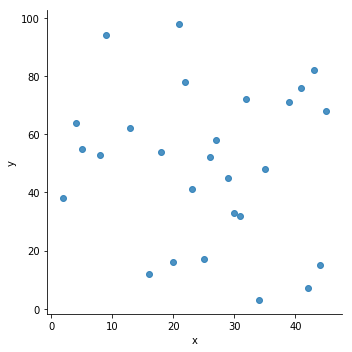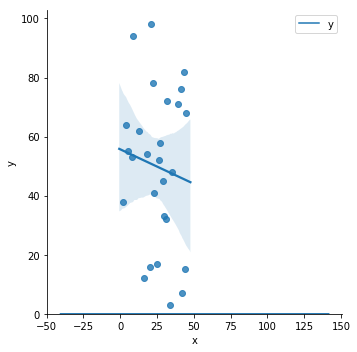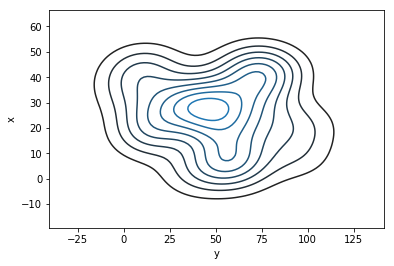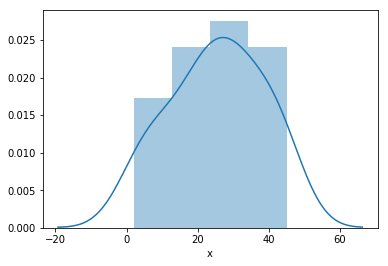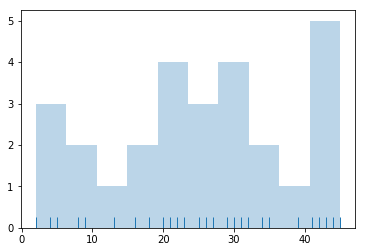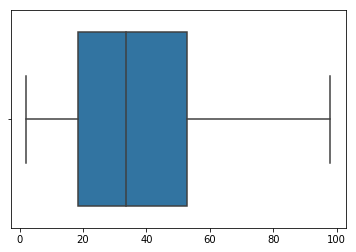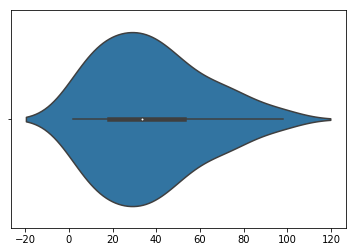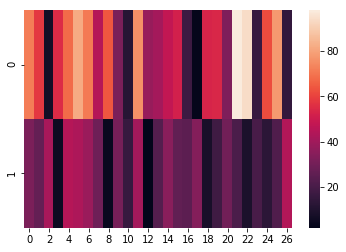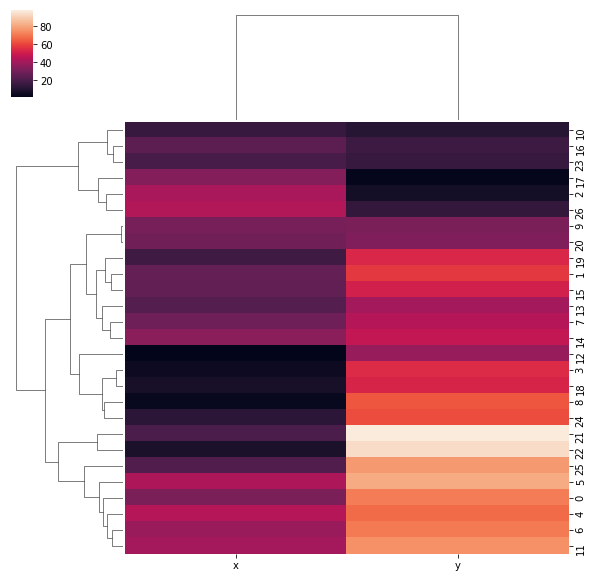#### Relevant Projects

##### Deep Learning with Keras in R to Predict Customer Churn
In this deep learning project, we will predict customer churn using Artificial Neural Networks and learn how to model an ANN in R with the keras deep learning package.

##### Ecommerce product reviews - Pairwise ranking and sentiment analysis
This project analyzes a dataset containing ecommerce product reviews. The goal is to use machine learning models to perform sentiment analysis on product reviews and rank them based on relevance. Reviews play a key role in product recommendation systems.

##### Solving Multiple Classification use cases Using H2O
In this project, we are going to talk about H2O and functionality in terms of building Machine Learning models.

##### Data Science Project on Wine Quality Prediction in R
In this R data science project, we will explore wine dataset to assess red wine quality. The objective of this data science project is to explore which chemical properties will influence the quality of red wines.

##### Walmart Sales Forecasting Data Science Project
Data Science Project in R-Predict the sales for each department using historical markdown data from the Walmart dataset containing data of 45 Walmart stores.

##### Ensemble Machine Learning Project - All State Insurance Claims Severity Prediction
In this ensemble machine learning project, we will predict what kind of claims an insurance company will get. This is implemented in python using ensemble machine learning algorithms.

##### Identifying Product Bundles from Sales Data Using R Language
In this data science project in R, we are going to talk about subjective segmentation which is a clustering technique to find out product bundles in sales data.

##### Learn to prepare data for your next machine learning project
Text data requires special preparation before you can start using it for any machine learning project.In this ML project, you will learn about applying Machine Learning models to create classifiers and learn how to make sense of textual data.

##### Machine Learning or Predictive Models in IoT - Energy Prediction Use Case
In this machine learning and IoT project, we are going to test out the experimental data using various predictive models and train the models and break the energy usage.

##### Natural language processing Chatbot application using NLTK for text classification
In this NLP AI application, we build the core conversational engine for a chatbot. We use the popular NLTK text classification library to achieve this.